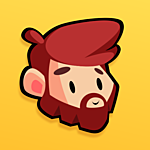# CommunityDoureyd
posted to: Creating the Unit

#### Get the Moving Left/Right Direction

I want to change my Unit Sprite Direction based on the current moving direction.

I just want to flip my Unit Horizontally and I would like to handle complex path like the one above : character moving from right to left then left to right.

Thanks!

•Nathan Lovato replied

Inside the process loop, I'd use code that sets the sprite's either scale or H flip property:

`func _process(delta: float) -> void:	var last_position = position	_path_follow.offset += move_speed * delta	var new_position = position		scale.x = sign(new_position.x - last_position.x)`

You'll have to fiddle with this to see how well it works. You might need to check for the case where the character is moving up to preserve the current direction. But the above should get you started.

•Doureyd replied
Solution

Thanks it's working with that :

```func _process(delta: float) -> void:	var last_position = _path_follow.position	_path_follow.offset += move_speed * delta
var new_position = _path_follow.position

var xDelta = int(round(new_position.x - last_position.x))
if xDelta != 0:
_sprite.scale.x = sign(xDelta)```
•Nathan Lovato replied

Sounds like something like that should work, yes!